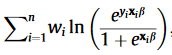# Adding weights to a Bernoulli distribution

I would like to create a weighted Bernoulli function in pyro (with known weights). As some of my observations are more trustworthy than others. The log-likelihood for LR:My question is how do I alter the likelihood of such Bernoulli function:

``````with pyro.plate('data'):
pyro.sample('y', dist.Bernoulli(logits=p), obs=y)
``````

Could I simply add to the power of the weights as shown below:

``````  with pyro.plate('data'):
pyro.sample('y', dist.Bernoulli(logits=torch.sign( p ) * torch.pow(torch.abs( p ), w)), obs=y)
``````

(with w being the weights)

My example runs, it only does not give me the desired results… Could someone please explain to me why this does not work or how I should implement it instead?

Hi @Helena.H,

I think you want `Bernoulli(logits=w * p)`. Note that `p` is kind of a confusing name for the `logits` arg since `p` so often denotes a probability as in `Bernoulli(probs=p)`, but logits is a log likelihood ratio:

``````probs = exp(logits) / (1 + exp(logits))
= 1 / (1 + exp(-logits))
logits = log(probs / (1 - probs))
``````

Good luck!

1 Like

You can also use

``````with pyro.plate('data'), pyro.poutine.scale(scale=w):
pyro.sample('y', dist.Bernoulli(logits=p), obs=y)
``````
1 Like